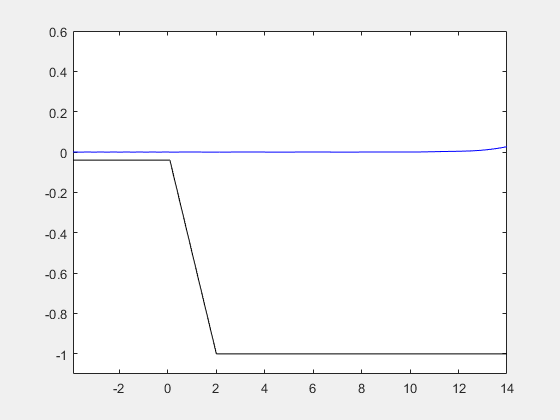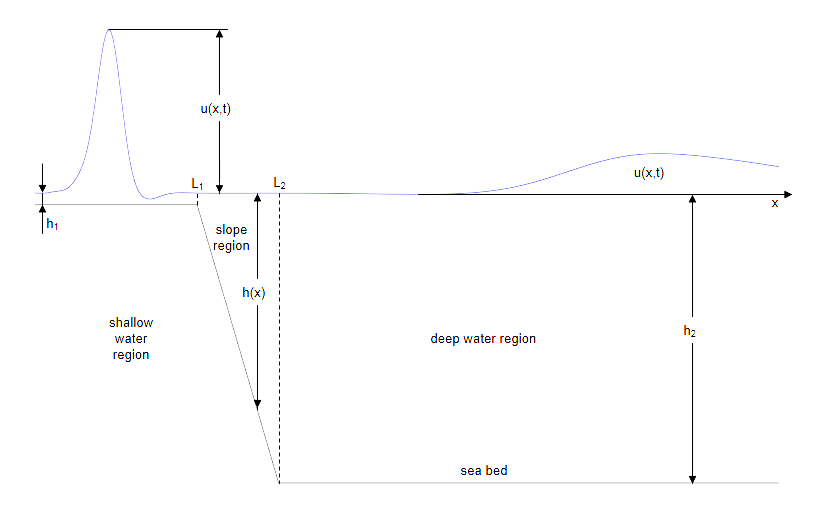Documentation

Solving Partial Differential Equations

This example simulates the tsunami wave phenomenon by using the Symbolic Math Toolbox™ to solve differential equations.

This simulation is a simplified visualization of the phenomenon, and is based on a paper by Goring and Raichlen .Mathematics of the Tsunami Model

A solitary wave (a soliton solution of the Korteweg-de Vries equation) travels at a constant speed from the right to the left along a canal of constant depth. This corresponds to a tsunami traveling over deep sea. At the left end of the canal, there is a slope simulating the continental shelf. After reaching the slope, the solitary wave begins to increase its height. When the water becomes very shallow, most of the wave is reflected back into the canal. However, a narrow but high peak of water arises at the end of the slope and proceeds with reduced speed in the original direction of the incident wave. This is the tsunami that finally hits the shore, causing disastrous destruction along the coastline. The speed of the wave nearing the shore is comparatively small. The wave eventually starts to break.Using linear dispersionless water theory, the height $u\left(x,t\right)$ of a free surface wave above the undisturbed water level in a one-dimensional canal of varying depth $h\left(x\right)$ is the solution of the following partial differential equation. (See .)

${u}_{tt}=g\phantom{\rule{0.16666666666666666em}{0ex}}\left(h\phantom{\rule{0.16666666666666666em}{0ex}}{u}_{x}{\right)}_{x}$

In this formula, subscripts denote partial derivatives, and $g=9.81m/{s}^{2}$ is the gravitational acceleration.

Consider a wave crossing a linear slope $h\left(x\right)$ from a region with the constant depth ${h}_{2}$ to a region with the constant depth ${h}_{1}\ll {h}_{2}$. The Fourier transformation with respect to $t$ turns the water wave partial differential equation to the following ordinary differential equation for the Fourier mode $u\left(x,t\right)=U\left(x,\omega \right)\phantom{\rule{0.16666666666666666em}{0ex}}{e}^{i\phantom{\rule{0.16666666666666666em}{0ex}}\omega \phantom{\rule{0.16666666666666666em}{0ex}}t}$.

$-{\omega }^{2}\phantom{\rule{0.16666666666666666em}{0ex}}U=g\phantom{\rule{0.16666666666666666em}{0ex}}\left(h\phantom{\rule{0.16666666666666666em}{0ex}}{U}_{x}{\right)}_{x}$

For the regions with constant depth $h$, the Fourier modes are traveling waves propagating in opposite directions with constant speed $c=\sqrt{g\phantom{\rule{0.16666666666666666em}{0ex}}h}$.

$u\left(x,t\right)={C}_{1}\left(\omega \right)\phantom{\rule{0.16666666666666666em}{0ex}}{e}^{i\phantom{\rule{0.16666666666666666em}{0ex}}\omega \phantom{\rule{0.16666666666666666em}{0ex}}\left(t+x/c\right)}+{C}_{2}\left(\omega \right)\phantom{\rule{0.16666666666666666em}{0ex}}{e}^{i\phantom{\rule{0.16666666666666666em}{0ex}}\omega \left(t-x/c\right)}$

The solution ${u}_{2}\left(x,t\right)={e}^{i\phantom{\rule{0.16666666666666666em}{0ex}}\omega \phantom{\rule{0.16666666666666666em}{0ex}}\left(t+x/{c}_{2}\right)}+R\left(\omega \right)\phantom{\rule{0.16666666666666666em}{0ex}}{e}^{i\phantom{\rule{0.16666666666666666em}{0ex}}\omega \phantom{\rule{0.16666666666666666em}{0ex}}\left(t-x/{c}_{2}\right)}$ for the deep water region is the superposition of two waves:

• a wave traveling to the left with constant speed ${c}_{2}=\sqrt{g\phantom{\rule{0.16666666666666666em}{0ex}}{h}_{2}}$

• a wave traveling to the right with an amplitude given by the frequency dependent reflection coefficient $R\left(\omega \right)$

This choice of ${u}_{2}$ satisfies the wave equation in the deep water region for any $R\left(\omega \right)$.

The solution ${u}_{1}\left(x,t\right)=T\left(\omega \right)\phantom{\rule{0.16666666666666666em}{0ex}}{e}^{i\phantom{\rule{0.16666666666666666em}{0ex}}\omega \left(t+x/{c}_{1}\right)}$ for the shallow water region is a transmitted wave traveling to the left with the constant speed ${c}_{1}=\sqrt{g\phantom{\rule{0.16666666666666666em}{0ex}}{h}_{1}}$. This choice of ${u}_{1}$ satisfies the wave equation in the shallow water region for any transmission coefficient $T\left(\omega \right)$.

For the transition region (the slope), use $u\left(x,t\right)=U\left(x,w\right)\phantom{\rule{0.16666666666666666em}{0ex}}{e}^{i\phantom{\rule{0.16666666666666666em}{0ex}}\omega \phantom{\rule{0.16666666666666666em}{0ex}}t}$.

Parameters and Solutions of the Tsunami Model in Symbolic Math Toolbox

Define the parameters of the tsunami model as follows. Disregard the dependency on the frequency $\omega$ in the following notations: $R=R\left(\omega \right)$, $T=T\left(\omega \right)$, $U\left(x\right)=U\left(x,\omega \right)$.

syms L H depthratio g positive
syms x t w T R U(x)

L1 = depthratio*L;
L2 = L;

h1 = depthratio*H;
h2 = H;
h(x) = x*H/L;

c1 = sqrt(g*h1);
c2 = sqrt(g*h2);

u(x,t)  = U(x)*exp(1i*w*t);
u1(x,t) = T*exp(1i*w*(t + x/c1));
u2(x,t) = exp(1i*w*(t + x/c2)) + R*exp(1i*w*(t - x/c2));

In the transition region over the linear slope, use dsolve to solve the ODE for the Fourier transform $U$ of $u$.

wavePDE(x,t) = diff(u,t,t) - g*diff(h(x)*diff(u,x),x);
slopeODE(x) = wavePDE(x,0);
U(x) = dsolve(slopeODE);

The solution $U$ is a complicated expression involving Bessel functions. It contains two arbitrary "constants" that depend on $\omega$.

Const = setdiff(symvar(U), sym([depthratio,g,H,L,x,w]))
Const = $\left(\begin{array}{cc}{C}_{1}& {C}_{2}\end{array}\right)$

For any Fourier mode, the overall solution must be a continuously differentiable function of $x$. Hence, the function values and the derivatives must match at the seam points ${L}_{1}$ and ${L}_{2}$. This provides four linear equations for $T$, $R$, and the two constants in $U$.

du1(x) = diff(u1(x,0),x);
du2(x) = diff(u2(x,0),x);
dU(x)  = diff(U(x),x);

eqs =  [ U(L1) == u1(L1,0), U(L2) == u2(L2,0),...
dU(L1) == du1(L1), dU(L2) == du2(L2)];
unknowns = [Const(1),Const(2),R,T];

Solve these equations.

[Cvalue1, Cvalue2, R, T] = solve(eqs, unknowns);

Substitute the results back into $R$, $T$, and $U$.

U(x) = subs(U(x), {Const(1),Const(2)}, {Cvalue1,Cvalue2});

You cannot directly evaluate the solution for $\omega =0$ because both numerator and denominator of the corresponding expressions vanish. Instead, find the low frequency limits of these expressions.

simplify(limit(U(x), w, 0))
ans =

$\frac{2}{\sqrt{\mathrm{depthratio}}+1}$

simplify(limit(R, w, 0))
ans =

$-\frac{\sqrt{\mathrm{depthratio}}-1}{\sqrt{\mathrm{depthratio}}+1}$

simplify(limit(T, w, 0))
ans =

$\frac{2}{\sqrt{\mathrm{depthratio}}+1}$

These limits are remarkably simple. They only depend on the ratio of the depth values defining the slope.

Substitute Symbolic Parameters with Numeric Values

For the following computations, use these numerical values for the symbolic parameters.

• Gravitational acceleration: $g=9.81\phantom{\rule{0.2777777777777778em}{0ex}}m/se{c}^{2}$

• Depth of the canal: $H=1\phantom{\rule{0.2777777777777778em}{0ex}}m$

• Depth ratio between the shallow and the deep regions: $depthratio=0.04$

• Length of the slope region: $L=2\phantom{\rule{0.2777777777777778em}{0ex}}m$

g = 9.81;
L = 2;
H = 1;
depthratio = 0.04;

h1 = depthratio*H;
h2 = H;

L1 = depthratio*L;
L2 = L;

c1 = sqrt(g*h1);
c2 = sqrt(g*h2);

Define the incoming soliton of amplitude $A$ traveling to the left with constant speed ${c}_{2}$ in the deep water region.

A = 0.3;
soliton = @(x,t) A.*sech(sqrt(3/4*g*A/H)*(x/c2+t)).^2;

Choose $Nt$ sample points for $t$. The time scale is chosen as a multiple of the (temporal) width of the incoming soliton. Store the corresponding discretized frequencies of the Fourier transform in $W$.

Nt =  64;
TimeScale = 40*sqrt(4/3*H/A/g);
W = [0, 1:Nt/2 - 1, -(Nt/2 - 1):-1]'*2*pi/TimeScale;

Choose $Nx$ sample points in $x$ direction for each region. Create sample points $X1$ for the shallow water region, $X2$ for the deep water region, and $X12$ for the slope region.

Nx = 100;
x_min = L1 - 4;
x_max = L2 + 12;
X12 = linspace(L1, L2, Nx);
X1  = linspace(x_min, L1, Nx);
X2  = linspace(L2, x_max, Nx);

Compute the Fourier transform of the incoming soliton on a time grid of $Nt$ equidistant sample points.

S = fft(soliton(-0.8*TimeScale*c2, linspace(0,TimeScale,2*(Nt/2)-1)))';
S = repmat(S,1,Nx);

Construct a traveling wave solution in the deep water region based on the Fourier data in $S$.

soliton = real(ifft(S .* exp(1i*W*X2/c2)));

Convert the Fourier modes of the reflected wave in the deep water region to numerical values over a grid in $\left(x,\omega \right)$ space. Multiply these values with the Fourier coefficients in $S$ and use the function ifft to compute the reflected wave in $\left(x,t\right)$ space. Note that the first row of the numeric data $R$ consists of NaN values because proper numerical evaluation of the symbolic data $R$ for $\omega =0$ is not possible. Define the values in the first row of $R$ as the low frequency limits.

R = double(subs(subs(vpa(subs(R)), w, W), x ,X2));
R(1,:) = double((1-sqrt(depthratio)) / (1+sqrt(depthratio)));
reflectedWave = real(ifft(S .* R .* exp(-1i*W*X2/c2)));

Use the same approach for the transmitted wave in the shallow water region.

T = double(subs(subs(vpa(subs(T)),w,W),x,X1));
T(1,:) = double(2/(1+sqrt(depthratio)));
transmittedWave = real(ifft(S .* T .* exp(1i*W*X1/c1)));

Also, use this approach for the slope region.

U12 = double(subs(subs(vpa(subs(U(x))),w,W),x,X12));
U12(1,:) = double(2/(1+sqrt(depthratio)));
U12 = real(ifft(S .* U12));

Plot the Solution

For a smoother animation, generate additional sample points using trigonometric interpolation along the columns of the plot data.

soliton = interpft(soliton, 10*Nt);
reflectedWave = interpft(reflectedWave, 10*Nt);
U12 = interpft(U12, 10*Nt);
transmittedWave = interpft(transmittedWave, 10*Nt);

Create an animated plot of the solution that shows-up in a separate figure window.

figure('Visible', 'on');
plot([x_min, L1, L2, x_max], [-h1, -h1, -h2, -h2], 'Color', 'black')
axis([x_min, x_max, -H-0.1, 0.6])
hold on

line1 = plot(X1,transmittedWave(1,:), 'Color', 'blue');
line12 = plot(X12,U12(1,:), 'Color', 'blue');
line2 = plot(X2,soliton(1,:) + reflectedWave(1,:), 'Color', 'blue');

for t = 2 : size(soliton, 1)*0.35
line1.YData = transmittedWave(t,:);
line12.YData = U12(t,:);
line2.YData = soliton(t,:) + reflectedWave(t,:);
pause(0.1)
endIn real life, tsunamis have a wavelength of hundreds of kilometers, often traveling at speeds of more than 500 km/hour. (Note that the average depth of the ocean is about 4 km, corresponding to a speed of $\sqrt{g\phantom{\rule{0.16666666666666666em}{0ex}}h}\approx \phantom{\rule{0.16666666666666666em}{0ex}}700\phantom{\rule{0.16666666666666666em}{0ex}}km/hour$.) Over deep sea, the amplitude is rather small, often about 0.5 m or less. When propagating onto the shelf, however, tsunamis increase their height dramatically: amplitudes of up to 30 m and more were reported.

One interesting phenomenon is that although tsunamis typically approach the coastline as a wave front extending for hundreds of kilometers perpendicular to the direction in which they travel, they do not cause uniform damage along the coast. At some points they cause disasters, whereas only moderate wave phenomena are observed at other places. This is caused by different slopes from the sea bed to the continental shelf. In fact, very steep slopes cause most of the tsunami to be reflected back into the region of deep water, whereas small slopes reflect less of the wave, transmitting a narrow but high wave carrying much energy.

Run the simulation for different values of $L$, which correspond to different slopes. The steeper the slope, the lower and less powerful the wave that is transmitted.

Note that this model ignores the dispersion and friction effects. On the shelf, the simulation loses its physical meaning. Here, friction effects are important, causing breaking of the waves.

References

 Derek G. Goring and F. Raichlen, Tsunamis - The Propagation of Long Waves onto a Shelf, Journal of Waterway, Port, Coastal and Ocean Engineering 118(1), 1992, pp. 41 - 63.

 H. Lamb, Hydrodynamics, Dover, 1932.

Mathematical Modeling with Symbolic Math Toolbox

Get examples and videos